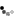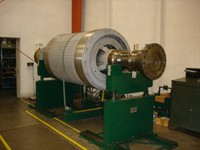Loading... Please wait...

• My Account
• Order Status

# Balancing Motor Armatures to ISO Grade G1

Posted

C D International, Inc.BALANCING ARMATURES TO QUALITY GRADE G1 OF ISO 1940/1 (ANSI S2.19-1975)

There are times when the balancing tolerance for an armature is specified based on the G number of the ISO or ANSI Standard. The following Tip will provide a quick way to calculate the G1.0 tolerance for armatures.If your balancing machine does not read out in units of weight (ounces, grams)  next weeks paper on CONVERTING AMPLITUDE READINGS(MILS, IN/SEC, G’S) TO UNBALANCE TOLERANCES (OZ-IN, GRAM-IN, GR-MM), will assist in using the Standard.

The EASA Standards for the Repair of Electrical Apparatus includes a section

(Section 2.7) on balancing. This reads as follows;“Dynamic Balancing should be to the level specified by the customer. In the absence of a requested level, dynamic balancing to balance quality grade G2.5 (ISO 1940/1) should enable the machine to meet final vibration limits as defined in 6.4.6.”

Reference: EASA Standards dated February, 1995, Section 2, Page 2.

More and more the customers are specifying a level and one of the most common is the G1 quality grade (ISO 1940/1). The following procedure presents an easy way to calculate the tolerance required for an armature based upon a customer specification of quality grade G1.0.

DISCUSSION

To understand and apply the referenced ISO Standard to rotor balancing requires a knowledge of three facts; the speed of the rotor, the dimensional shape of the rotor and the weight of the rotor.Applying the Standard to motor armatures is simplified since the speed is always known and the dimensional shape is generally symmetrical.This allows for a simplified approach for easy calculation of the tolerance based upon weight.

For reference only, the general formulas for calculating unbalance tolerances to the ISO Standard are as follows;

(a) Acceptable Level of Unbalance in ounce inches = 6.015 x G x W/N

(b) Acceptable Level of Unbalance in gram inches= 170.5 x G x W/N

(c) Acceptable Level of Unbalance in gram mm=9549 x G x W/N

where G is the balance quality grade from the ISO Standard

W is the weight of the rotor in pounds (a & b) or Kgs (c)

N is the operating speed of the rotor

and the acceptable level of unbalance calculated is total for the rotor.

Since most motor armatures are symmetrical (the total unbalance can be divided by 2 for per plane corrections) and the RPM is always known, we can easily calculate the tolerance based on weight.

As an example, to calculate ounce inches of unbalance for a 3600 RPM symmetrical armature;

Acceptable level on balance per plane in ounce inches  = 6.015 x G x W/N

2

= 6.015 x 1.0 x W/3600

2

= .00167 x W / 2

= .00084 x W

For any 3600 RPM motor armature, the balance tolerance per plane can be determined from this formula by simply knowing the weight of the armature.

The following chart will show the simplified formulas for 3600, 1800, 1200, 900 and 600 RPM as well as several examples.

 FORMULA 3600 RPM 1800 RPM 1200 RPM 900 RPM 600 RPM Allowable Unbalance per plane (oz - in) = .00084 x W .00167 x W .00251 x W .00334 x W .00501 x W Allowable Unbalance per plane (gm - in) = . 02368 x W .04736 x W .07104 x W .09472 x W .14208 x W

W = Weight of the Rotor in pounds

EXAMPLES

Unbalance Tolerance per plane in both ounce inches and gram inches.

 Rotor Weight in Pounds 3600 RPM 1800 RPM 1200 RPM 900 RPM 600 RPM oz-in/ plane gm-in/ plane oz-in/ plane gm-in/ plane oz-in/ plane gm-in/ plane oz-in/ plane gm-in/ plane oz-in/ plane gm-in/ plane 250 0.21 5.92 .4175 11.84 .6275 17.76 0.835 23.68 1.253 35.52 500 0.42 11.84 .835 23.68 1.255 35.52 1.67 47.36 2.505 71.04 1000 0.84 23.68 1.67 47.36 2.51 71.04 3.34 94.72 5.01 142.1 2000 1.68 47.36 3.34 94.72 5.02 142.1 6.68 189.4 10.02 284.2 2500 2.10 59.20 4.175 118.4 6.275 177.6 8.35 236.8 12.53 355.2 4000 3.36 94.72 6.68 189.4 10.04 284.2 13.36 378.9 20.04 568.3 5000 4.20 118.4 8.35 236.8 12.55 355.2 16.7 473.6 25.05 710.4 7500 6.30 177.6 12.53 355.2 18.83 532.8 25.05 710.4 37.58 1066 10000 8.40 236.8 16.70 473.6 25.10 710.4 33.4 947.2 50.10 1421 15000 12.60 355.2 25.05 710.4 37.65 1066 50.1 1421 75.15 2131

PROCEDURE

STEP 1.

Select the proper formula above based on the RPM to balance a rotor to G1.0 from the ISO Standard.

STEP 2.

Weigh the rotor and calculate the proper tolerance per plane from the formula.

STEP 3.

When the proper tolerance has been calculated, divide the tolerance by the radius at which weight will be added.

EXAMPLE:The unbalance tolerance for a 1000 pound, 3600 RPM rotor is

23.68 gram inches per plane.The radius to the balance ring is 10 inches.

Weight = unbalance tolerance / radius = 23.68 / 10= 2.4 grams per plane

STEP 4.

Balance the rotor until the unbalance tolerance has been met.

Although C D International, Inc believes reasonable efforts have been made to ensure the accuracy of the information contained in this document, it may include inaccuracies or typographical errors and may be changed or updated without notice. It is intended for discussion and educational purposes only and is provided "as is" without warranty of any kind and reliance on any information presented is at your own risk.